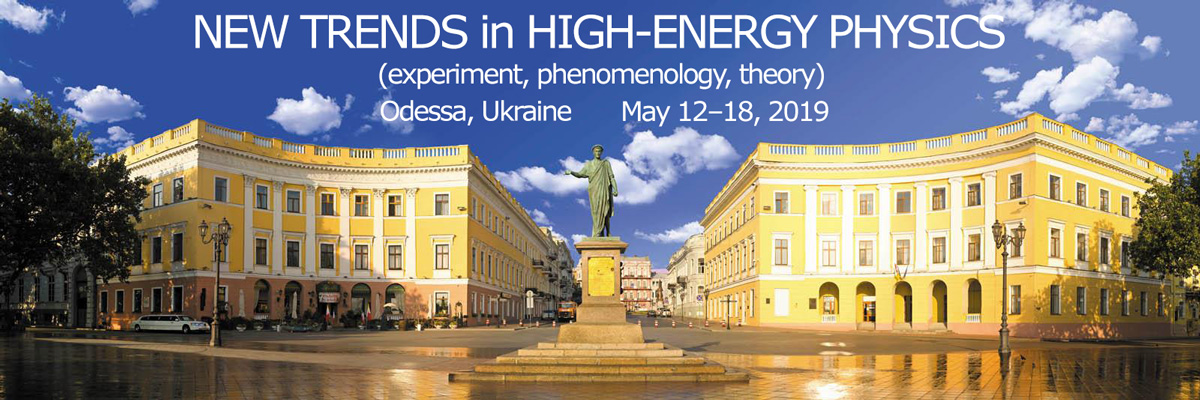#New Trends in High-Energy Physics

12-18 May 2019
Europe/Kiev timezone

## On particle-like models in vacuum space-times with torsion and nonmetricity tensors

Not scheduled
25m

24 Genuezkaya St., Odessa 65009, Ukraine

### Speaker

Vyacheslav Olyeynik (Odessa I.I.Mechnikov National University)

### Description

In tensor analysis we deal with tensor objects that are different in nature: scalars, vectors, tensors of rank n. When the basis (four non-collinear non-coplanar basis vectors) is introduced at each point of space-time, all these objects may be treated as the n-polylinear combinations of the basis vectors with scalar coefficients. This means that the variation of any Lagrangian with tensor fields can be represented as the variation over scalar fields (with some special properties) and over the vector fields which define the basis. Moreover, if we introduce a holonomic basis, the non-gradient parts of the vectors of the conjugate basis can also be considered as fields that need to be introduced into the model. For each of the non-gradient fields included in the model, we can write equations of the Maxwell type with corresponding currents. In the case of vacuum models with real field functions, all such currents contribute to the distribution of mass density and its fluxes in the space-time.
We present some results of studies of particle-like models with Lagrangians containing only bilinear combinations of first derivatives of all fields defining the space-time geometry.

### Primary author

Vyacheslav Olyeynik (Odessa I.I.Mechnikov National University)

### Presentation Materials

There are no materials yet.